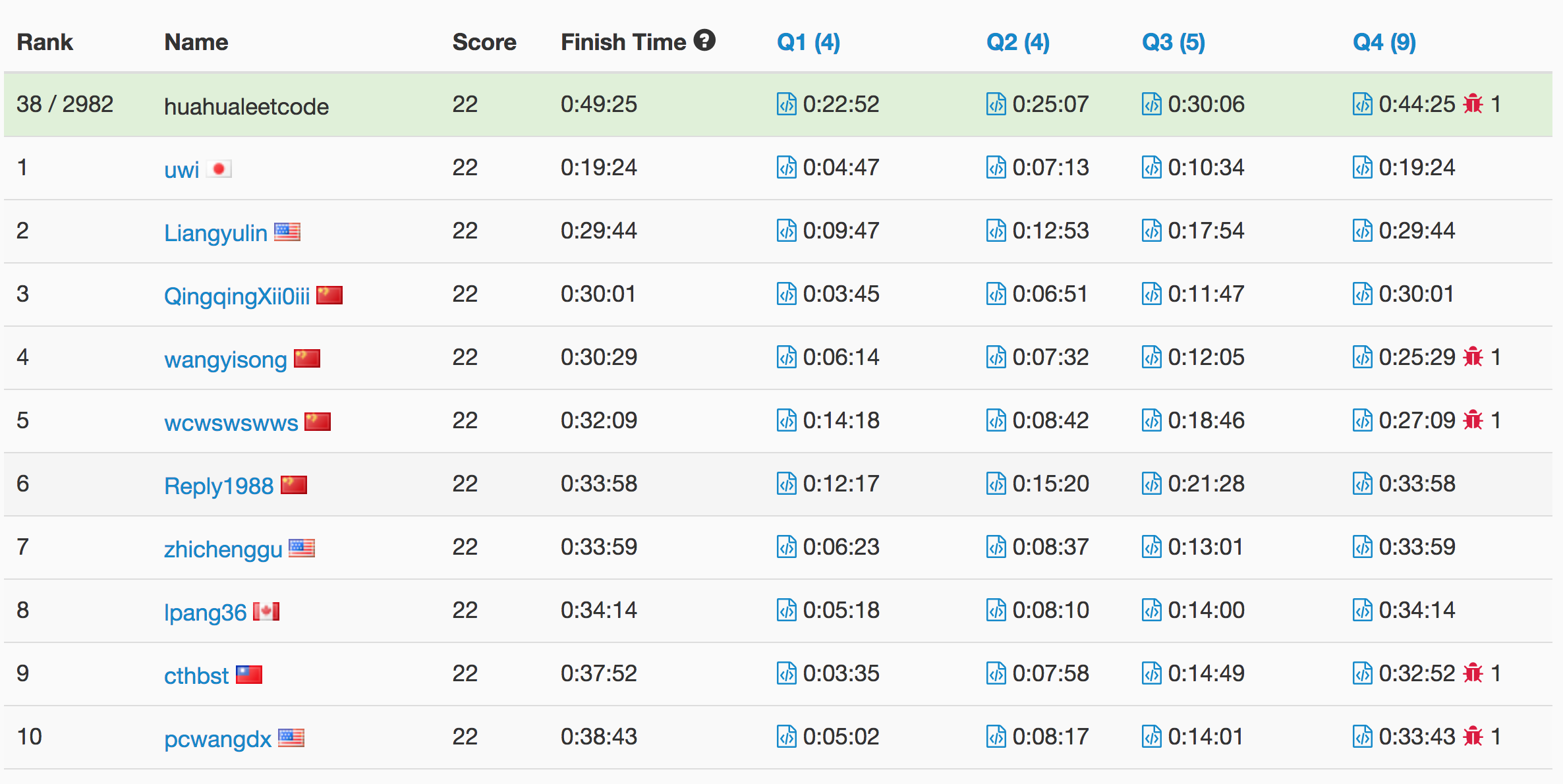Press "Enter" to skip to content

# Problem

https://leetcode.com/problems/valid-square/description/

Given the coordinates of four points in 2D space, return whether the four points could construct a square.

The coordinate (x,y) of a point is represented by an integer array with two integers.

Example:

Input: p1 = [0,0], p2 = [1,1], p3 = [1,0], p4 = [0,1]
Output: True


Note:

1. All the input integers are in the range [-10000, 10000].
2. A valid square has four equal sides with positive length and four equal angles (90-degree angles).
3. Input points have no order.

# Solution

Time complexity: O(1)

Space complexity: O(1)

C++

Write a bash script to calculate the frequency of each word in a text file words.txt.

For simplicity sake, you may assume:

• words.txt contains only lowercase characters and space ' ' characters.
• Each word must consist of lowercase characters only.
• Words are separated by one or more whitespace characters.

For example, assume that words.txt has the following content:

the day is sunny the the
the sunny is is


Your script should output the following, sorted by descending frequency:

the 4
is 3
sunny 2
day 1


Note:
Don’t worry about handling ties, it is guaranteed that each word’s frequency count is unique.

# Ranking

Full list here# Problem

We are to write the letters of a given string S, from left to right into lines. Each line has maximum width 100 units, and if writing a letter would cause the width of the line to exceed 100 units, it is written on the next line. We are given an array widths, an array where widths is the width of ‘a’, widths is the width of ‘b’, …, and widths is the width of ‘z’.

Now answer two questions: how many lines have at least one character from S, and what is the width used by the last such line? Return your answer as an integer list of length 2.

Example :
Input:
widths = [10,10,10,10,10,10,10,10,10,10,10,10,10,10,10,10,10,10,10,10,10,10,10,10,10,10]
S = "abcdefghijklmnopqrstuvwxyz"
Output: [3, 60]
Explanation:
All letters have the same length of 10. To write all 26 letters,
we need two full lines and one line with 60 units.

Example :
Input:
widths = [4,10,10,10,10,10,10,10,10,10,10,10,10,10,10,10,10,10,10,10,10,10,10,10,10,10]
S = "bbbcccdddaaa"
Output: [2, 4]
Explanation:
All letters except 'a' have the same length of 10, and
"bbbcccdddaa" will cover 9 * 10 + 2 * 4 = 98 units.
For the last 'a', it is written on the second line because
there is only 2 units left in the first line.
So the answer is 2 lines, plus 4 units in the second line.


Note:

• The length of S will be in the range [1, 1000].
• S will only contain lowercase letters.
• widths is an array of length 26.
• widths[i] will be in the range of [2, 10].

# Solution: Simulation

Time Complexity: O(n)

Space Complexity: O(1)

# Problem

International Morse Code defines a standard encoding where each letter is mapped to a series of dots and dashes, as follows: "a" maps to ".-""b" maps to "-...""c" maps to "-.-.", and so on.

For convenience, the full table for the 26 letters of the English alphabet is given below:

[".-","-...","-.-.","-..",".","..-.","--.","....","..",".---","-.-",".-..","--","-.","---",".--.","--.-",".-.","...","-","..-","...-",".--","-..-","-.--","--.."]

Now, given a list of words, each word can be written as a concatenation of the Morse code of each letter. For example, “cab” can be written as “-.-.-….-“, (which is the concatenation “-.-.” + “-…” + “.-“). We’ll call such a concatenation, the transformation of a word.

Return the number of different transformations among all words we have.

Example:
Input: words = ["gin", "zen", "gig", "msg"]
Output: 2
Explanation:
The transformation of each word is:
"gin" -> "--...-."
"zen" -> "--...-."
"gig" -> "--...--."
"msg" -> "--...--."

There are 2 different transformations, "--...-." and "--...--.".


Note:

• The length of words will be at most 100.
• Each words[i] will have length in range [1, 12].
• words[i] will only consist of lowercase letters.

# Solution: HashTable

Time Complexity: O(n)

Space Complexity: O(n)

C++

Mission News Theme by Compete Themes.